Documentation

## Identify Nonlinear Black-Box Models Using System Identification App

### Introduction

#### Objectives

Estimate and validate nonlinear models from single-input/single-output (SISO) data to find the one that best represents your system dynamics.

After completing this tutorial, you will be able to accomplish the following tasks using the System Identification app:

• Import data objects from the MATLAB® workspace into the app.

• Estimate and validate nonlinear models from the data.

• Plot and analyze the behavior of the nonlinearities.

#### Data Description

This tutorial uses the data file `twotankdata.mat`, which contains SISO time-domain data for a two-tank system, shown in the following figure.

Two-Tank System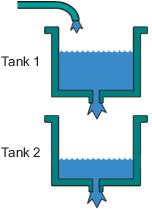In the two-tank system, water pours through a pipe into Tank 1, drains into Tank 2, and leaves the system through a small hole at the bottom of Tank 2. The measured input u(t) to the system is the voltage applied to the pump that feeds the water into Tank 1 (in volts). The measured output y(t) is the height of the water in the lower tank (in meters).

Based on Bernoulli's law, which states that water flowing through a small hole at the bottom of a tank depends nonlinearly on the level of the water in the tank, you expect the relationship between the input and the output data to be nonlinear.

`twotankdata.mat` includes 3000 samples with a sample time of 0.2 s.

### What Are Nonlinear Black-Box Models?

#### Types of Nonlinear Black-Box Models

You can estimate nonlinear discrete-time black-box models for both single-output and multiple-output time-domain data. You can choose from two types of nonlinear, black-box model structures:

• Nonlinear ARX models

• Hammerstein-Wiener models

### Note

You can estimate Hammerstein-Wiener black-box models from input/output data only. These models do not support time-series data, where there is no input.

For more information on estimating nonlinear black-box models, see Nonlinear Model Identification.

#### What Is a Nonlinear ARX Model?

A nonlinear ARX model consists of model regressors and a nonlinearity estimator. The nonlinearity estimator comprises both linear and nonlinear functions that act on the model regressors to give the model output. This block diagram represents the structure of a nonlinear ARX model in a simulation scenario.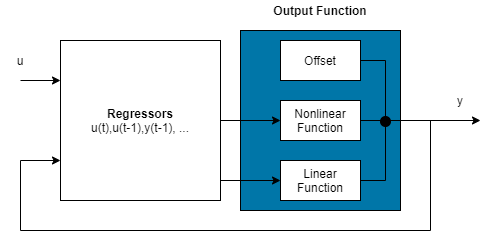The software computes the nonlinear ARX model output y in two stages:

1. It computes regressor values from the current and past input values and past output data.

In the simplest case, regressors are delayed inputs and outputs, such as u(t-1) and y(t-3). These kind of regressors are called standard regressors. You specify the standard regressors using the model orders and delay. For more information, see Nonlinear ARX Model Orders and Delay. You can also specify custom regressors, which are nonlinear functions of delayed inputs and outputs. For example, u(t-1)*y(t-3). To create a set of polynomial type regressors, use `polyreg`.

By default, all regressors are inputs to both the linear and the nonlinear function blocks of the nonlinearity estimator. You can choose a subset of regressors as inputs to the nonlinear function block.

2. It maps the regressors to the model output using the nonlinearity estimator block. The nonlinearity estimator block can include linear and nonlinear blocks in parallel. For example:

`$F\left(x\right)={L}^{T}\left(x-r\right)+d+g\left(Q\left(x-r\right)\right)$`

Here, x is a vector of the regressors, and r is the mean of the regressors x. ${L}^{T}\left(x\right)+d$ is the output of the linear function block and is affine when d ≠ 0. d is a scalar offset. $g\left(Q\left(x-r\right)\right)$ represents the output of the nonlinear function block. Q is a projection matrix that makes the calculations well conditioned. The exact form of F(x) depends on your choice of the nonlinearity estimator. You can select from available nonlinearity estimators, such as tree-partition networks, wavelet networks, and multilayer neural networks. You can also exclude either the linear or the nonlinear function block from the nonlinearity estimator.

When estimating a nonlinear ARX model, the software computes the model parameter values, such as L, r, d, Q, and other parameters specifying g.

Resulting nonlinear ARX models are `idnlarx` objects that store all model data, including model regressors and parameters of the nonlinearity estimator. For more information about these objects, see Nonlinear Model Structures.

#### What Is a Hammerstein-Wiener Model?

This block diagram represents the structure of a Hammerstein-Wiener model: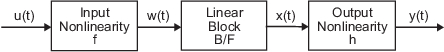Where,

• f is a nonlinear function that transforms input data u(t) as w(t) = f(u(t)).

w(t), an internal variable, is the output of the Input Nonlinearity block and has the same dimension as u(t).

• B/F is a linear transfer function that transforms w(t) as x(t) = (B/F)w(t).

x(t), an internal variable, is the output of the Linear block and has the same dimension as y(t).

B and F are similar to polynomials in a linear Output-Error model. For more information about Output-Error models, see What Are Polynomial Models?.

For ny outputs and nu inputs, the linear block is a transfer function matrix containing entries:

`$\frac{{B}_{j,i}\left(q\right)}{{F}_{j,i}\left(q\right)}$`

where j = `1,2,...,ny` and i = `1,2,...,nu`.

• h is a nonlinear function that maps the output of the linear block x(t) to the system output y(t) as y(t) = h(x(t)).

Because f acts on the input port of the linear block, this function is called the input nonlinearity. Similarly, because h acts on the output port of the linear block, this function is called the output nonlinearity. If your system contains several inputs and outputs, you must define the functions f and h for each input and output signal. You do not have to include both the input and the output nonlinearity in the model structure. When a model contains only the input nonlinearity f, it is called a Hammerstein model. Similarly, when the model contains only the output nonlinearity h, it is called a Wiener model.

The software computes the Hammerstein-Wiener model output y in three stages:

1. Compute w(t) = f(u(t)) from the input data.

w(t) is an input to the linear transfer function B/F.

The input nonlinearity is a static (memoryless) function, where the value of the output a given time t depends only on the input value at time t.

You can configure the input nonlinearity as a sigmoid network, wavelet network, saturation, dead zone, piecewise linear function, one-dimensional polynomial, or a custom network. You can also remove the input nonlinearity.

2. Compute the output of the linear block using w(t) and initial conditions: x(t) = (B/F)w(t).

You can configure the linear block by specifying the orders of numerator B and denominator F.

3. Compute the model output by transforming the output of the linear block x(t) using the nonlinear function h as y(t) = h(x(t)).

Similar to the input nonlinearity, the output nonlinearity is a static function. You can configure the output nonlinearity in the same way as the input nonlinearity. You can also remove the output nonlinearity, such that y(t) = x(t).

Resulting models are `idnlhw` objects that store all model data, including model parameters and nonlinearity estimators. For more information about these objects, see Nonlinear Model Structures.

### Preparing Data

Load sample data in `twotankdata.mat` by typing the following command in the MATLAB Command Window:

```load twotankdata ```

This command loads the following two variables into the MATLAB Workspace browser:

• `u` is the input data, which is the voltage applied to the pump that feeds the water into Tank 1 (in volts).

• `y` is the output data, which is the water height in Tank 2 (in meters).

#### Creating iddata Objects

System Identification Toolbox™ data objects encapsulate both data values and data properties into a single entity. You can use the System Identification Toolbox commands to conveniently manipulate these data objects as single entities.

Use the following commands to create two `iddata` data objects, `ze` and `zv`, where `ze` contains data for model estimation and `zv` contains data for model validation. `Ts` is the sample time.

```Ts = 0.2; % Sample time is 0.2 sec z = iddata(y,u,Ts); % First 1000 samples used for estimation ze = z(1:1000); % Remaining samples used for validation zv = z(1001:3000);```

To view the properties of the `iddata` object, use the `get` command. For example:

`get(ze)`

MATLAB software returns the following data properties and values:

``` Domain: 'Time' Name: '' OutputData: [1000x1 double] y: 'Same as OutputData' OutputName: {'y1'} OutputUnit: {''} InputData: [1000x1 double] u: 'Same as InputData' InputName: {'u1'} InputUnit: {''} Period: Inf InterSample: 'zoh' Ts: 0.2000 Tstart: 0.2000 SamplingInstants: [1000x0 double] TimeUnit: 'seconds' ExperimentName: 'Exp1' Notes: {} UserData: []```

To modify data properties, use dot notation. For example, to assign channel names and units that label plot axes, type the following syntax in the MATLAB Command Window:

```% Set time units to minutes ze.TimeUnit = 'sec'; % Set names of input channels ze.InputName = 'Voltage'; % Set units for input variables ze.InputUnit = 'V'; % Set name of output channel ze.OutputName = 'Height'; % Set unit of output channel ze.OutputUnit = 'm'; % Set validation data properties zv.TimeUnit = 'sec'; zv.InputName = 'Voltage'; zv.InputUnit = 'V'; zv.OutputName = 'Height'; zv.OutputUnit = 'm';```

To verify that the `InputName` property of `ze` is changed, type the following command:

`ze.inputname`

### Tip

Property names, such as `InputName`, are not case sensitive. You can also abbreviate property names that start with `Input` or `Output` by substituting `u` for `Input` and `y` for `Output` in the property name. For example, `OutputUnit` is equivalent to `yunit`.

#### Starting the System Identification App

To open the System Identification app, type the following command in the MATLAB Command Window:

`systemIdentification`

The default session name, `Untitled`, appears in the title bar.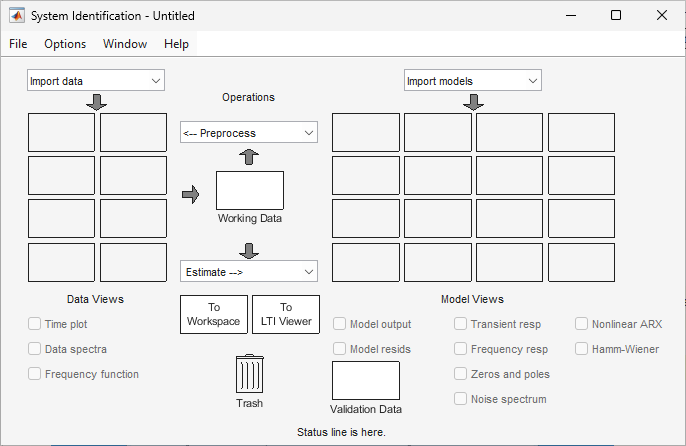#### Importing Data Objects into the System Identification App

You can import the data objects into the app from the MATLAB workspace.

You must have already created the data objects, as described in Creating iddata Objects, and opened the app, as described in Starting the System Identification App.

To import data objects:

1. In the System Identification app, select Import data > Data object.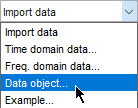This action opens the Import Data dialog box.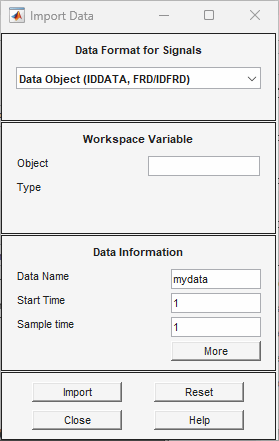2. Enter `ze` in the Object field to import the estimation data. Press Enter.

This action enters the object information into the Import Data fields.

3. Click to add the icon named `ze` to the System Identification app.

4. In the Import Data dialog box, type `zv` in the Object field to import the validation data. Press Enter.

5. Click to add the icon named `zv` to the System Identification app.

6. In the Import Data dialog box, click .

7. In the System Identification app, drag the validation data zv icon to the Validation Data rectangle. The estimation data ze icon is already designated in the Working Data rectangle.

Alternatively, right-click the `zv` icon to open the Data/model Info dialog box. Select the Use as Validation Data check-box. Click and then to add `zv` to the Validation Data rectangle.

The System Identification app now resembles the following figure.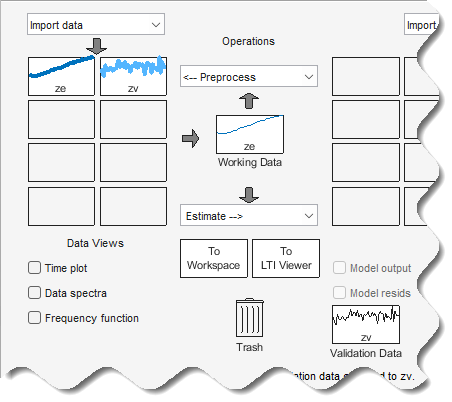### Estimating Nonlinear ARX Models

#### Estimating a Nonlinear ARX Model with Default Settings

In this portion of the tutorial, you estimate a nonlinear ARX model using default model structure and estimation options.

You must have already prepared the data, as described in Preparing Data. For more information about nonlinear ARX models, see What Is a Nonlinear ARX Model?

1. In the System Identification app, select Estimate > Nonlinear models.This action opens the Nonlinear Models dialog box.The Configure tab is already open and the default Model type is ```Nonlinear ARX```.

In the Regressors tab, the Input Channels and Output Channels have Delay set to `1` and No. of Terms set to `2`. The model output y(t) is related to the input u(t) via the following nonlinear autoregressive equation:

`$y\left(t\right)=f\left(y\left(t-1\right),y\left(t-2\right),u\left(t-1\right),u\left(t-2\right)\right)$`

f is the nonlinearity estimator selected in the Nonlinearity drop-down list of the Model Properties tab, and is `Wavelet Network` by default. The number of units for the nonlinearity estimator is set to Select automatically and controls the flexibility of the nonlinearity—more units correspond to a more flexible nonlinearity.

2. Click .

This action adds the model `nlarx1` to the System Identification app, as shown in the following figure.The Nonlinear Models dialog box displays a summary of the estimation information in the Estimate tab. The Fit (%) is the mean square error between the measured data and the simulated output of the model: 100% corresponds to a perfect fit (no error) and 0% to a model that is not capable of explaining any of the variation of the output and only the mean level.### Note

Fit (%) is computed using the estimation data set, and not the validation data set. However, the model output plot in the next step compares the fit to the validation data set.

3. In the System Identification app, select the Model output check box.

This action simulates the model using the input validation data as input to the model and plots the simulated output on top of the output validation data.The Best Fits area of the Model Output plot shows that the agreement between the model output and the validation-data output.

#### Plotting Nonlinearity Cross-Sections for Nonlinear ARX Models

Perform the following procedure to view the shape of the nonlinearity as a function of regressors on a Nonlinear ARX Model plot.

1. In the System Identification app, select the Nonlinear ARX check box to view the nonlinearity cross-sections.

By default, the plot shows the relationship between the output regressors `Height(t-1)` and `Height(t-2)`. This plot shows a regular plane in the following figure. Thus, the relationship between the regressors and the output is approximately a linear plane.2. In the Nonlinear ARX Model Plot window, set Regressor 1 to `Voltage(t-1)`. Set Regressor 2 to `Voltage(t-2)`. Click .

The relationship between these regressors and the output is nonlinear, as shown in the following plot.3. To rotate the nonlinearity surface, select Style > Rotate 3D and drag the plot to a new orientation.

4. To display a 1-D cross-section for Regressor 1, set Regressor 2 to `none`, and click . The following figure shows the resulting nonlinearity magnitude for Regressor 1, which represents the time-shifted voltage signal, `Voltage(t-1)`.#### Changing the Nonlinear ARX Model Structure

In this portion of the tutorial, you estimate a nonlinear ARX model with specific input delay and nonlinearity settings. Typically, you select model orders by trial and error until you get a model that produces an accurate fit to the data.

You must have already estimated the nonlinear ARX model with default settings, as described in Estimating a Nonlinear ARX Model with Default Settings.

1. In the Nonlinear Models dialog box, click the Configure tab, and click the Regressors tab.

2. For the `Voltage` input channel, double-click the corresponding Delay cell, enter `3`, and press Enter.

This action updates the Resulting Regressors list to show `Voltage(t-3)` and `Voltage(t-4)` — terms with a minimum input delay of three samples.

3. Click .

This action adds the model `nlarx2` to the System Identification app and updates the Model Output window to include this model. The Nonlinear Models dialog box displays the new estimation information in the Estimate tab.4. In the Nonlinear Models dialog box, click the Configure tab, and select the Model Properties tab.

5. In the Number of units in nonlinear block area, select Enter, and type `6`. This number controls the flexibility of the nonlinearity.6. Click .

This action adds the model `nlarx3` to the System Identification app. It also updates the Model Output window, as shown in the following figure.#### Selecting a Subset of Regressors in the Nonlinear Block

You can estimate a nonlinear ARX model that includes only a subset of standard regressors that enter as inputs to the nonlinear block. By default, all standard and custom regressors are used in the nonlinear block. In this portion of the tutorial, you only include standard regressors.

You must have already specified the model structure, as described in Changing the Nonlinear ARX Model Structure.

1. In the Nonlinear Models dialog box, click the Configure tab, and select the Regressors tab.

2. Click the button to open the Model Regressors dialog box.3. Clear the following check boxes:

• Height(t-2)

• Voltage(t-3)

Click .

This action excludes the time-shifted `Height(t-2)` and `Voltage(t-3)` from the list of inputs to the nonlinear block.

4. Click .

This action adds the model `nlarx4` to the System Identification app. It also updates the Model Output window.

#### Specifying a Previously-Estimated Model with Different Nonlinearity

You can estimate a series of nonlinear ARX models by making systematic variations to the model structure and base each new model on the configuration of a previously estimated model. In this portion of the tutorial, you estimate a nonlinear ARX model that is similar to an existing model (`nlarx3`), but with a different nonlinearity.

1. In the Nonlinear Models dialog box, select the Configure tab. Click Initialize. This action opens the Initial Model Specification dialog box.

2. In the Initial Model list, select `nlarx3`. Click .3. Click the Model Properties tab.

4. In the Nonlinearity list, select ```Sigmoid Network```.

5. In the Number of units in nonlinear block field, type `6`.6. Click .

This action adds the model `nlarx5` to the System Identification app. It also updates the Model Output plot, as shown in the following figure.#### Selecting the Best Model

The best model is the simplest model that accurately describes the dynamics.

To view information about the best model, including the model order, nonlinearity, and list of regressors, right-click the model icon in the System Identification app.

### Estimating Hammerstein-Wiener Models

#### Estimating Hammerstein-Wiener Models with Default Settings

In this portion of the tutorial, you estimate nonlinear Hammerstein-Wiener models using default model structure and estimation options.

You must have already prepared the data, as described in Preparing Data. For more information about nonlinear ARX models, see What Is a Hammerstein-Wiener Model?

1. In the System Identification app, select Estimate > Nonlinear models to open the Nonlinear Models dialog box.

2. In the Configure tab, select `Hammerstein-Wiener` in the Model type list.The I/O Nonlinearity tab is open. The default nonlinearity estimator is `Piecewise Linear` with `10` units for Input Channels and Output Channels, which corresponds to `10` breakpoints for the piecewise linear function.

3. Select the Linear Block tab to view the model orders and input delay.By default, the model orders and delay of the linear output-error (OE) model are nb=2, nf=3, and nk=1.

4. Click .

This action adds the model `nlhw1` to the System Identification app.

5. In the System Identification app, select the Model output check box.

This action simulates the model using the input validation data as input to the model and plots the simulated output on top of the output validation data.

The Best Fits area of the Model Output window shows the agreement between the model output and the validation-data output.

#### Plotting the Nonlinearities and Linear Transfer Function

You can plot the input/output nonlinearities and the linear transfer function of the model on a Hammerstein-Wiener plot.

1. In the System Identification app, select the Hamm-Wiener check box to view the Hammerstein-Wiener model plot.

The plot displays the input nonlinearity, as shown in the following figure.2. Click the yNL rectangle in the top portion of the Hammerstein-Wiener Model Plot window.

The plot updates to display the output nonlinearity.3. Click the Linear Block rectangle in the top portion of the Hammerstein-Wiener Model Plot window.

The plot updates to display the step response of the linear transfer function.4. In the Choose plot type list, select `Bode`. This action displays a Bode plot of the linear transfer function.#### Changing the Hammerstein-Wiener Model Input Delay

In this portion of the tutorial, you estimate a Hammerstein-Wiener model with a specific model order and nonlinearity settings. Typically, you select model orders and delays by trial and error until you get a model that produces a satisfactory fit to the data.

You must have already estimated the Hammerstein-Wiener model with default settings, as described in Estimating Hammerstein-Wiener Models with Default Settings.

1. In the Nonlinear Models dialog box, click the Configure tab, and select the Linear Block tab.

2. For the `Voltage` input channel, double-click the corresponding Input Delay (nk) cell, change the value to `3`, and press Enter.

3. Click .

This action adds the model `nlhw2` to the System Identification app and the Model Output window is updated to include this model, as shown in the following figure.The Best Fits area of the Model Output window shows the quality of the `nlhw2` fit.

#### Changing the Nonlinearity Estimator in a Hammerstein-Wiener Model

In this portion of the example, you modify the default Hammerstein-Wiener model structure by changing its nonlinearity estimator.

### Tip

If you know that your system includes saturation or dead-zone nonlinearities, you can specify these specialized nonlinearity estimators in your model. `Piecewise Linear` and `Sigmoid Network` are nonlinearity estimators for general nonlinearity approximation.

1. In the Nonlinear Models dialog box, click the Configure tab.

2. In the I/O Nonlinearity tab, for the `Voltage` input, click the Nonlinearity cell, and select ```Sigmoid Network``` from the list. Click the corresponding No. of Units cell and set the value to `20`.3. Click .

This action adds the model `nlhw3` to the System Identification app. It also updates the Model Output window, as shown in the following figure.4. In the Nonlinear Models dialog box, click the Configure tab.

5. In the I/O Nonlinearity tab, set the `Voltage` input Nonlinearity to `Wavelet Network`. This action sets the No. of Units to be determined automatically, by default.

6. Set the `Height` output Nonlinearity to ```One-dimensional Polynomial```.7. Click .

This action adds the model `nlhw4` to the System Identification app. It also updates the Model Output window, as shown in the following figure.#### Selecting the Best Model

The best model is the simplest model that accurately describes the dynamics.

In this example, the best model fit was produced in Changing the Nonlinearity Estimator in a Hammerstein-Wiener Model.

##### Support